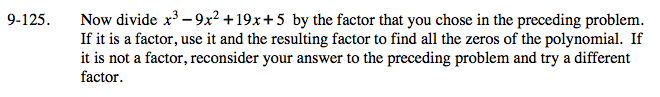Home > A2C > Chapter 9 > Lesson 9.3.1 > Problem9-125

9-125.

Now divide x3 − 9x2 + 19x + 5 by the factor that you chose in the preceding problem. If it is a factor, use it and the resulting factor to find all the zeros of the polynomial. If it is not a factor, reconsider your answer to the preceding problem and try a different factor. Homework Help ✎Divide x3 − 9x2 + 19x + 5 by x − 5.

Use a multiplication rectangle to help you set it up.

(x − 5)(x2 − 4x − 1)

Now find all factors and solutions.

$x=5, 2\pm\sqrt{5}$